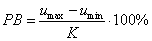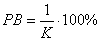﻿ 20-sim webhelp > Library > Signal > Control > PID Control > Proportional Control

# Proportional Control

## Gain

When we talk about the proportional action of a controller, we generally refer to the proportional gain. The action means that the controller moves in proportion to the error between setpoint (SP) and process output (PV):

controller output = K*error = K*(SP - PV)

where the gain is denoted by the parameter K. Many terms have been used by different manufacturers to designate this action. It has been called proportional gain, gain, throttling band, sensitivity and proportional band.

## Proportional Band

In practice, the controller output is limited, either by its own limitations or by the limitations of the corresponding actuator. Let umax and umin denote the minimum and maximum output of the controller. The proportional band of the controller is then defined as:In the ideal case, a controller can have an unlimited output. The proportional band (PB) is then defined as:This definition of proportional band is often used instead of the controller gain. The value is expressed in percent (%).

## Direct Acting / Reverse Acting

Suppose a process with a controller output CV and a process output PV is in steady state and kept to a certain setpoint SP. For proportional control the following equations can be found:

PV = P(CO)

CV = K*error = K*(SP - PV)

where P is the process function that yields a measured variable as a result of the controller output. If the controller output decreases as the measured variable increases the controller is said to be direct acting. If the controller output increases as the measured variable increases, then it is called reverse acting. In other words, the controller is direct acting if the gain K is positive and reverse acting if the gain K is negative.

This is not only valid for proportional control, but for all PID controllers in the 20-sim library. If you need a reverse acting controller, simply use a negative gain.

## Offset

If you look at the equation for the proportional gain:

CV = K*error = K*(SP - PV)

you will notice that there has to be an error to produce a controller output. This means that with proportional control only, there will always be a small offset between the setpoint and the measured variable. To remove this offset, integral control has to be used.